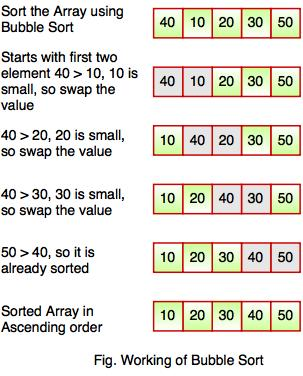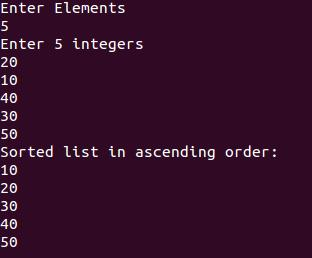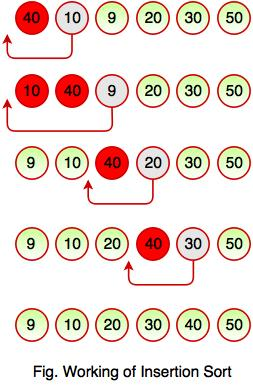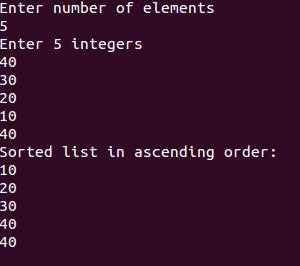# Sorting in Data Structure

## What is Sorting?

Sorting is a process of ordering or placing a list of elements from a collection in some kind of order. It is nothing but storage of data in sorted order. Sorting can be done in ascending and descending order. It arranges the data in a sequence which makes searching easier.

For example, suppose we have a record of employee. It has following data:

Employee No.
Employee Name
Employee Salary
Department Name

Here, employee no. can be takes as key for sorting the records in ascending or descending order. Now, we have to search a Employee with employee no. 116, so we don't require to search the complete record, simply we can search between the Employees with employee no. 100 to 120.

## Sorting Techniques

Sorting technique depends on the situation. It depends on two parameters.

1. Execution time of program that means time taken for execution of program.
2. Space that means space taken by the program.

Sorting techniques are differentiated by their efficiency and space requirements.

Sorting can be performed using several techniques or methods, as follows:

1. Bubble Sort
2. Insertion Sort
3. Selection Sort
4. Quick Sort
5. Heap Sort

#### 1. Bubble Sort

• Bubble sort is a type of sorting.
• It is used for sorting 'n' (number of items) elements.
• It compares all the elements one by one and sorts them based on their values.

•• The above diagram represents how bubble sort actually works. This sort takes O(n2) time. It starts with the first two elements and sorts them in ascending order.
• Bubble sort starts with first two elements. It compares the element to check which one is greater.
• In the above diagram, element 40 is greater than 10, so these values must be swapped. This operation continues until the array is sorted in ascending order.

#### Example: Program for Bubble Sort

#include <stdio.h>
void bubble_sort(long [], long);

int main()
{
long array, n, c, d, swap;
printf("Enter Elements\n");
scanf("%ld", &n);
printf("Enter %ld integers\n", n);
for (c = 0; c < n; c++)
scanf("%ld", &array[c]);
bubble_sort(array, n);
printf("Sorted list in ascending order:\n");
for ( c = 0 ; c < n ; c++ )
printf("%ld\n", array[c]);
return 0;
}
void bubble_sort(long list[], long n)
{
long c, d, t;
for (c = 0 ; c < ( n - 1 ); c++)
{
for (d = 0 ; d < n - c - 1; d++)
{
if (list[d] > list[d+1])
{
/* Swapping */
t         = list[d];
list[d]   = list[d+1];
list[d+1] = t;
}
}
}
}

Output:#### 2. Insertion Sort

• Insertion sort is a simple sorting algorithm.
• This sorting method sorts the array by shifting elements one by one.
• It builds the final sorted array one item at a time.
• Insertion sort has one of the simplest implementation.
• This sort is efficient for smaller data sets but it is insufficient for larger lists.
• It has less space complexity like bubble sort.
• It requires single additional memory space.
• Insertion sort does not change the relative order of elements with equal keys because it is stable.

•• The above diagram represents how insertion sort works. Insertion sort works like the way we sort playing cards in our hands. It always starts with the second element as key. The key is compared with the elements ahead of it and is put it in the right place.
• In the above figure, 40 has nothing before it. Element 10 is compared to 40 and is inserted before 40. Element 9 is smaller than 40 and 10, so it is inserted before 10 and this operation continues until the array is sorted in ascending order.

#### Example: Program for Insertion Sort

#include <stdio.h>

int main()
{
int n, array, c, d, t;
printf("Enter number of elements\n");
scanf("%d", &n);
printf("Enter %d integers\n", n);
for (c = 0; c < n; c++)
{
scanf("%d", &array[c]);
}
for (c = 1 ; c <= n - 1; c++)
{
d = c;
while ( d > 0 && array[d] < array[d-1])
{
t               = array[d];
array[d]    = array[d-1];
array[d-1] = t;
d--;
}
}
printf("Sorted list in ascending order:\n");
for (c = 0; c <= n - 1; c++)
{
printf("%d\n", array[c]);
}
return 0;
}

Output: